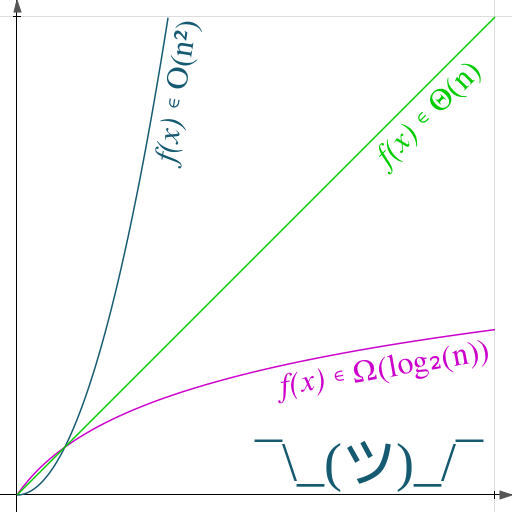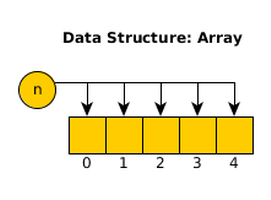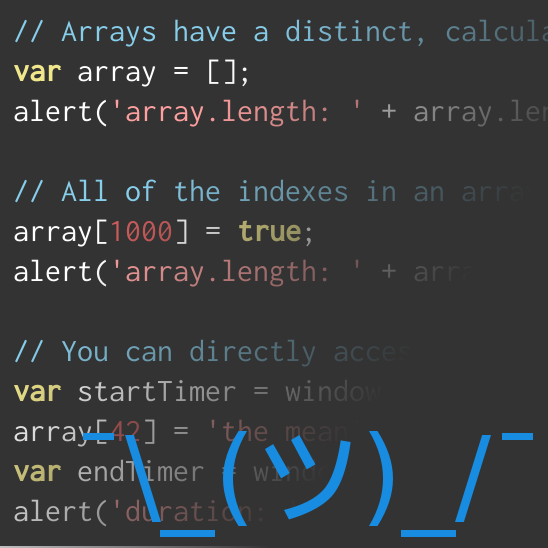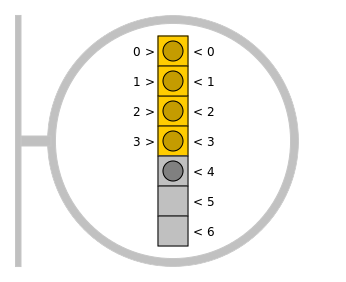## Asymptotic Notation: A PrimerHow well will this code run?

If you're like me, you probably ask yourself this question fairly often. It's always disappointing to spend a lot of time on a project only to realize that you've created a bottleneck that is wrestling your program's performance through the floor. If only there were a way to predict a program's performance before writing any code, right?

### Counting Instructions

There's something intuitive about the idea that we should be able to extrapolate performance from the number of instructions that get executed. Every instruction a processor executes costs some time, so the more instructions that a processor executes the more time a process will take to run. Unfortunately, this requires an intimate knowledge of the compiler or interpreter, as well as a the architecture of the systems running the program.

This kind of optimization would only be meaningful within a verry narrow set of system parameters, and would only be useful if memory or processing power are at a premium. Since most of us aren't puttingrovers on Mars, however, we are going to need a more generic solution.

### Asymptotic Behavior

Simply put, asymptotic notation is a technique for describing how an algorithm behaves as the size of the input grows. Suppose that you have two piles of rice. After every second, you add one grain of rice to the first pile, and then you double the size of the second pile. Given an infinite amount of time, both stacks of rice would be infinitely large. But would they be the same size?

Mathematically, the pile of rice that doubles every second will be a much, much larger infinity, and we can prove it (warning: math).

#### Doing the Math

Let's assume that k is the starting size of the pile of rice, and x is equal to the number of seconds that have elapsed since we started. We can determine how many grains of rice are in the first pile with the formula f(x) = k + x — the number of grains in the pile is equalto the starting size plus one grain for each second that has passed. Likewise, we can determine how many grains of rice are in the second pile with the formula g(x) = k · 2x — the number of grains in the pile is equal to the starting size of the pile, doubled every second.

Before we get into the weeds with trying to prove this on paper, let's assume that each pile started with 1 grain of rice and see what that looks like on a graph:The pile that doubles every second — g(x) — grows at a much faster rate than the pile that we add one grain at a time. This trend persists regardless of the starting size of the piles.

That's great, Steve, but what does rice have to do with computing?

Nothing, but the math does. This math lets us figure out how much of a resource (in this case, rice) is consumed by a function as the input (in this case, time) grows. If we were to apply this logic to computing, we would usually measure some computing resource (time, memory, storage, etc.) proportional to some variable input (the length of an array, the magnitude of an integer, etc.).

There's one major difference between counting grains of rice and measuring performance in a computer that changes how we must treat our math: there are a lot of factors that affect computation speed in a computer that wouldn't affect the number of grains of rice. What kind of processor is executing the code? What operating system? How is the operating system allocating resources? There are a host of factors that can affect the actual execution time, so there often isn't any value in trying to determine the precise resource consumption of a function.

But all is not lost! Just because we can't determine the exact number of milliseconds it takes to execute a set of commands doesnt' mean that our estimates are useless. The first step is to remove the constants from the expressions: f(x) ≈ x and g(x) ≈ 2x. If we say that these functions return the same results, and that their values correspond to resource consumption, then we can extrapolate that the function with the smaller growth rate will have higher performance.

Now we that we have the theory, let's see the application.

#### Practical Application

Typically, we will measure asymptotes as a function of the size of the input, where the result is the amount of a resource that is consumed. Usually, that resource is either time or an abstract concept of complexity, but it could be anything. Consider the following code snippet:

/**
* Basic Fibonnaci Sequence Generator
* 0, 1, 1, 2, 3, 5, ...
*
* @param {integer} x   x'th number from the sequence
* @return the value of the x'th number from the sequence
*/
function fib(x)
{
if (!x || x <= 0) {
return 0;
}
if (x <= 1) {
return 1;
}
return fib(x - 2) + fib(x - 1);
}

The basic Fibonnaci Sequence Generator is a relatively simple function that performs like absolute crap. I encourage you to try it, but don't do it in a browser you aren't willing to force-close, because the resource consumption of this function is exponential. It takes roughly twice as long to calculate the value of fib(5) as it took to calculate the value of fib(4). Let's compare this to a memoized version of thefunction:

/**
* Memoized Fibonnaci Sequence Generator
* 0, 1, 1, 2, 3, 5, ...
*
* @param {integer} x   x'th number from the sequence
* @return the value of the x'th number from the sequence
*/
var betterFib = (function() {
var _memo = {};
return function betterFib(x) {
if (!x || x <= 0) {
return 0;
}
if (x <= 1) {
return 1;
}
if ('undefined' === typeof _memo[x]) {
_memo[x] = betterFib(x - 2) + betterFib(x - 1);
}
return _memo[x];
};
})();

By caching the results of this function, we can ensure that any value of x must only be calculated once. This should give us a pretty decent speed bump, but how would we explain that decision to someone? Sure, in this instance it's pretty easy, but what if we were trying to decide between something more complicated, or if we're relying on code that we don't maintain?

We use asymptotic notation.

### Different Notations & What they Mean

There are several different notationst hat are used to describe asymptotic behaviors, and each one describes a different aspect of that behavior. In a nutshell, Big-Oh represents the worst-case behavior of a function, Big-Omega represents the best-case behavior of a function, and Big-Theta represents the way a function always behaves. Definitions aren't useful unless you understand what they mean, though, so let's apply them to our functions fib and betterFib.

#### Big-Oh (Ο)

Big-Oh is typically used to describe the upper-boundary of a function. That doesn't always mean that it's your worst-case behavior; but if we are measuring resource consumption, then it represents the behavior of the maximum resource consumption of a function. Big-Oh also doesn't imply that this is how a function will perform. It's an informational tool to explain how the "upper-bound" of resource consumption will behave proportional to the size of the input. If we look back to fib and betterFib, we have already determined our Big-Oh values:

For the function fib, any value x > 1 causes fib to execute twice as many times as the previous value of x. Since we care about asymptotic behavior, we can represent this by stating that fib executes at Ο(2n).

For the function betterFib, the memoization ensures that we only need to calculate a value once. Any value x > 1 still causes betterFib to execute itself twice, but the value of betterFib(x - 1) includes the value of betterFib(x - 2). The net result of memoization is that betterFib executes in linear time — Ο(n) — for any value of x.

Big-Oh is the most commonly used asymptotic notation in computing. Since Big-Oh measures the way "poor performance" behaves, it's especially useful for reducing user frustration. As a general rule, avoiding "poor performance" is more important than maximizing "good performance"; and if you aren't convinced of the veracity of that statement, think back to the last time you became consciously aware that your internet was fast vs. the last time you became consciously aware that your internet was slow.

#### Big-Omega (Ω)

Big-Omega is less commonly used in computing, but is still occasionally useful. Big Omega looks just like Big-Oh, but uses the capital letter Omega (Ω) instead of Omicron (Ο). Where Big-Oh is used to describe the "worst case" behavior of a function, Big-Omega describes the lower boundary behavior of a function. Again, this doesn't describe how a function will perform, but it provides some insight into the lowest consumption you can expect from a function. This can be useful in instances where you are tie-breaking between two functions that have similar Big-Oh performance. Let's examine fib and betterFib to determine their Big-Omega behaviors.

The function fib has no variance whatsoever. Regardless of how many times the function is run, it will always perform terribly. Since the function has the same asymptotic behavior, we can represent it the same way: Ω(2n).

The function betterFib, however, uses memoization to improve performance. If you ran betterFib(1000) once, it would execute at Ο(n). If you ran betterFib again with any input x ≤ 1000, it would return the value from the _memo object without recursing. This means that the "best case" you can get out of this function is constant time — Ω(1).

This is an even broader difference than we saw from Big-Oh.

#### Big-Theta (Θ)

Big-Theta is less commonly used than Big-Oh, but still important to recognize. Big-Theta is used to describe functions in which the upper-bound behavior and the lower-bound behavior are the same. The function fib will execute at both Ο(2n) and Ω(2n). This means that fib also performs at Θ(2n). By comparison, the memoization of betterFib causes it to execute at Ο(n) and Ω(1); so it has no Big-Theta value.

### Summary

When approaching a problem, it's helpful to understand how different solutions may impact the performance of your program. Even seemingly simple optimizations can seriously improve the performance of a piece of software in ways that may not always be obvious. Likewise, some optimizations may be wasting your time without providing a net benefit to your execution time. Asymptotic notation provides a framework to explain how different functions behave with large input values. Understanding how to read and derive Big-Oh, Big-Omega, and Big-Theta will improve your ability to write high-performance code, to integrate other people's code into your projects, and to explain to potential third-parties how to best integrate your code into their projects. Sure, it takes a little extra work, but I promise that it's worth it.

### Related Blog PostsData Structure: Array